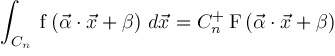Planet Quantum News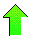Contents News View

 Jun 1, 2005 - An n-Dimensional Integral Formula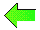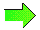This note (PDF version) discusses a generalization of some formulas in "A Heuristic for Exact Calculation of n-Dimensional (n+1)-Parametric Integrals of Some Elementary and Special Functions" by Vladimir V. Bondarenko.  The following simple theorem can be used in combination with the more interesting results appearing in the next two sections. Theorem.Suppose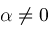and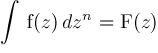. Then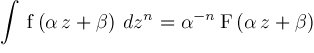. An n-Dimensional F Integral Theorem.Assume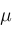is not a negative integer and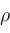is a positive integer.  Then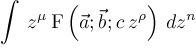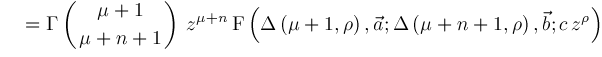Comment.Since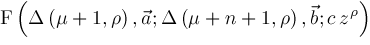for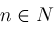are generated by a finite dimensional basis over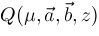, the indefinite integrals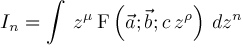satisfy a linear recurrence relation.  This linear recurrence relation can be explicitly computed. Example.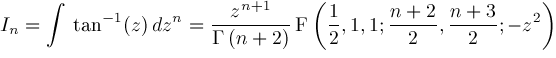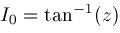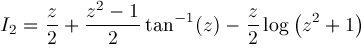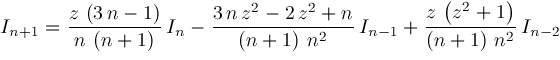Example.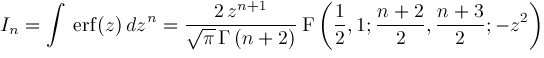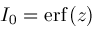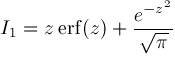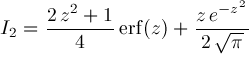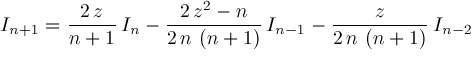An n-Dimensional G Integral Theorem.Assumeis a positive integer. Then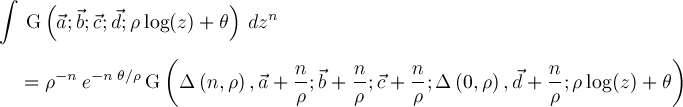Definite Integrals Definition.Let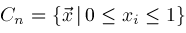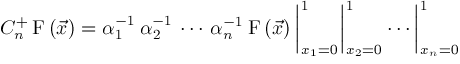Theorem.Assume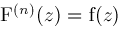.  Then